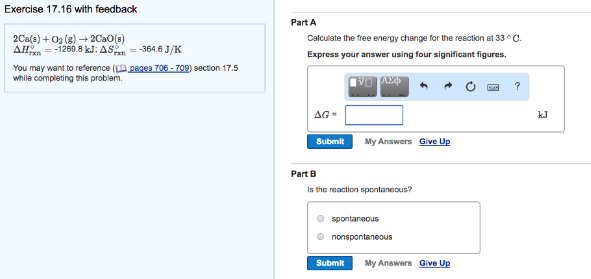# Problem: 2Ca (s) + O2 (g) → 2CaO (s) ΔH°rxn = -1269.8 kJ; ΔS°rxn = - 364.6 J/K Part ACalculate the free energy change for the reaction at 33°C. Express your answer using four significant figures.Part B Is the reaction spontaneous?                                  (i) spontaneous                                  (ii) nonspontaneous

###### FREE Expert Solution
91% (254 ratings)###### Problem Details

2Ca (s) + O2 (g) → 2CaO (s)

ΔH°rxn = -1269.8 kJ; ΔS°rxn = - 364.6 J/K

Part A

Calculate the free energy change for the reaction at 33°C.

Part B

Is the reaction spontaneous?

(i) spontaneous

(ii) nonspontaneous